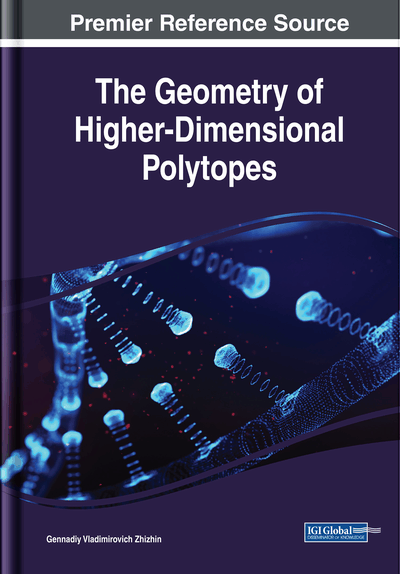# The Number of Symmetry Transformation of Convex Regular Polytopes in the n - Space

DOI: 10.4018/978-1-5225-6968-8.ch003
Available
\$37.50
No Current Special Offers

## Abstract

The number of symmetry transformations of regular polytopes of dimension n (n - cubes, n - simplexes, n - cross polytopes) are considered, using symmetry transformation of their facets. In this chapter, it is investigated how a certain symmetry transformation of the facet leads to the transformation of the symmetry of the polytope, under the condition of continuity of the polytope as a whole. It is established that the number of symmetry transformations of a regular n - polytope is equal to the product of the number of symmetry transformations of the facet of the corresponding polytope by the number of facets in this polytope.
Chapter Preview
Top

## The Number Of Symmetry Transformation Of Regular N – Simplex

A regular simplex consists of five regular tetrahedrons (Stringham, 1880), whose faces are regular triangles. Therefore, the symmetry transformations of a regular simplex are linked inextricably with the symmetry transformations of a regular tetrahedron and a regular triangle as the faces of a regular simplex. The regular triangle (Figure 1) has six symmetry operations that reunite the triangle with itself:

3 mirror reflection operations with respect to the bisectors m, n, k of angles at the vertices a, b, c; 2 turns of the triangle relative to the center of the triangle, for example, clockwise to the angles, and; the identity transformation that leaves the triangle in place.

The remaining movements of the triangle, which combine it with oneself (rotations to angles multipleclockwise and counterclockwise, combinations of rotations with mirror reflections) reduce to the symmetry transformations already listed. To each of the six symmetry transformations there corresponds a certain permutation of three vertices a, b, c. Since the number of these permutations is equal to 3!, the total number of these permutations is 6. It is essential that under the indicated symmetry transformations all elements of dimension less than the dimension of the triangle appearing in the triangle go over into one another or into themselves. The vertices pass into each other or into themselves, but also sides of triangle A, B, C cross one another or into themselves. In this case, the triangle does not break into its constituent parts. The number of permutation of the sides also equal to 3!. Moreover, to any permutation of sides there corresponds some permutation of vertices. For example, permutation of sidescorresponds to a permutation of vertices, and permutation of sidescorresponds to permutation of vertices.When the side is repainted by itself (the first case side C), it rotates 180 degrees.

## Key Terms in this Chapter

The Symmetry Transformation of the Figure: Figure movement, combining the figure with itself.

N – Cube: Convex polytope of dimension n , all vertices have connections with an edges to n other vertices of this polytope.

Facet: Face with dimension n – 1 in the polytope with dimension n .

N – Simplex: Convex polytope of dimension n , all vertices of which have connections with an edge to all other vertices of the vertices of this polytope.

N – Cross – Polytope: A convex polytope of dimension n in which every two vertices opposite to the center of the polytope have no connection by an edge, and the remaining vertices are joined by edges.

## Complete Chapter List

Search this Book:
Reset